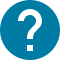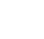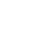学部前期課程
HOME 学部前期課程 全学自由研究ゼミナール（PEAK)(Single-variable calculus)　(Single-variable calculus)

UTASにアクセスできない方は、担当教員または部局教務へお問い合わせ下さい。

# 全学自由研究ゼミナール（PEAK)(Single-variable calculus)　(Single-variable calculus)

Single-variable calculus
In this course, we study the differentiation and integration of a function of one-variable (which is also called one-variable function or single-variable function). Intuitively, differential means the slope of the tangent line of a function at a given point, and integral measures the area bounded by the graph of the function and x-axis over a given interval. Both concepts need the concept “limit”, so the first part of this course will contain the definition of a function, limit of a sequence, continuous function and related topics. In the second part of this course, we study the differential of a function, the definition, calculation techniques, properties and applications. In the third part, we define the definite and indefinite integral of a function, study the fundamental theorem of calculus: the connection between differentiation and integration, and introduce the calculation techniques. Power series will also be introduced. In the end, we discuss how to solve some simple differential equations.

コース名

50831
CAS-TC1200S1

A1 A2

2

NO

1. Sequence, limit, continuous function 2. The derivative of a function and higher-order derivatives 3. Taylor's theorem and extreme values 4. Taylor series 5. Integral of a function 6. the fundamental theorem 7. Integration methods 8. Differential equations

Blackboard lectures (except for the first week when the lecture will be given over Zoom). Each class extends for 105 minutes.

Final exam, plus some quiz given during lectures.

Mathematics I ①, described on this page, is the first portion of the Calculus course provided for PEAK, with second portion, Mathematics I ②, to be provided in 2024 S semester. In the lectures to be given in Mathematics I ②, the students are assumed to have acquired familiarity with the materials taught in Mathematics I ①.